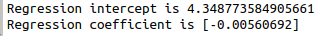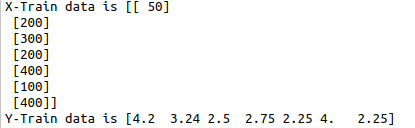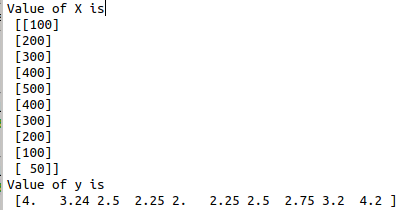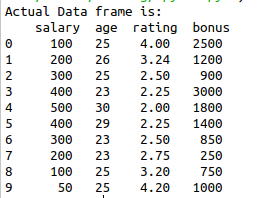• #5, First Floor, 4th Street Dr. Subbarayan Nagar Kodambakkam, Chennai-600 024 Landmark : Samiyar Madam
• pro@slogix.in
• +91- 81240 01111

### How to split data set into train and test in simple linear regression using python?

###### Description

To split data set into train and test in a simple linear regression model in python.

###### Process

Import regression model from scikit-learn library.

Import train_test_split from scikit-learn library.

Define independent(X) and dependent(Y) variable.

Define the training data set from data sample.

###### Sample Code

#import needed library

import matplotlib.pyplot as plt

import pandas as pd

import numpy as np

#import linear model and train_test_split

from sklearn.model_selection import train_test_split

from sklearn.linear_model import LinearRegression

data={‘salary’:[100,200,

300,400,500,400,300,200,100,50],

‘age’:[25,26,

25,23,30,29,23,23,25,25],’rating’:

[4,3.24,2.5,2.25,2,2.25,2.5,2.75,3.2,4.2],

‘bonus’:[2500,1200,900,

3000,1800,1400,850,250,750, 1000]}

#create the data frame

df=pd.DataFrame(data)

print(“Actual Data frame is:\n”,df)

#Define x(independent variable)

X = df.iloc[:, :1].values

print(“Value of X is\n”,X)

#Define y(dependent variable)

y = df.iloc[:,2].values

print(“Value of y is\n”,y)

#declare the train data set

X_train, X_test, y_train, y_test = train_test_split

(X, y, test_size=0.3, random_state=0)

#build linear model

regressor = LinearRegression()

#pass the X-train and y_train

t=regressor.fit(X_train, y_train)

print(“X-Train data is”,X_train)

print(“Y-Train data is”,y_train)

print(“Regression intercept is”,regressor.intercept_)

print(“Regression coefficient is”,regressor.coef_)

###### Screenshots